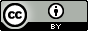Wen, Y.; Su, L. M.; Qin, W. C.; Fu, L.; He, J.; Zhao, Y. H. Linear and non-linear relationships between soil sorption and hydrophobicity: Model, validation and influencing factors. Chemosphere 2012, 86, 634–640.

QsarDB content

Property logKoc: Logarithm of soil sorption coefficient i

Compounds: 701 | Models: 3 | Predictions: 6

Eq1: Linear correlation with logP i

Regression model (regression)

Open in:QDB Explorer QDB Predictor

Name Type n

R2

σ

Training set training 594 0.750 0.604
Validation set external validation 107 0.811 0.634
Eq2: Non-linear regression model i

Regression model (regression)

Open in:QDB Explorer QDB Predictor

Name Type n

R2

σ

Training set training 594 0.785 0.546
Validation set external validation 107 0.809 0.625
Eq3: Non-linear regression model with correction factors i

Regression model (regression)

Open in:QDB Explorer QDB Predictor

Name Type n

R2

σ

Training set training 594 0.826 0.497
Validation set external validation 107 0.809 0.625

Citing

When using this QDB archive, please cite (see details) it together with the original article:

 Title: Wen, Y.; Su, L. M.; Qin, W. C.; Fu, L.; He, J.; Zhao, Y. H. Linear and non-linear relationships between soil sorption and hydrophobicity: Model, validation and influencing factors. Chemosphere 2012, 86, 634–640. Abstract: The hydrophobic parameter represented by the octanol/water partition coefficient (logP) is commonly used to predict the soil sorption coefficient (Koc). However, a simple non-linear relationship between logKoc and logP has not been reported in the literature. In the present paper, soil sorption data for 701 compounds was investigated. The results show that logKoc is linearly related to logP for compounds with logP in the range of 0.5–7.5 and non-linearly related to logP for the compounds in a wide range of logP. A non- linear model has been developed between logKoc and log P for a wide range of compounds in the training set. This model was validated in terms of average error (AE), average absolute error (AAE) and root-mean squared error (RMSE) by using an external test set with 107 compounds. Nearly the same predictive capacity was observed in comparison with existing models. However, this non-linear model is simple, and uses only one parameter. The best model developed in this paper is a non-linear model with six cor- rection factors for six specific classes of compounds. This model can well predict logKoc for 701 diverse compounds with AAE = 0.37. The reasons for systemic deviations in these groups may be attributed to the difference of sorption mechanism for hydrophilic/polar compounds, low solubility for highly hydrophobic compounds, hydrolysis of esters in solution, volatilization for volatile compounds and highly experimental errors for compounds with extremely high or low sorption coefficients. URI: http://hdl.handle.net/10967/159 http://dx.doi.org/10.15152/QDB.159 Date: 2015-05-28
﻿

Files in this item

Name Description Format Size View
2011C634.qdb.zip Linear and non-linear regression models for logKoc application/x-zip 304.6Kb View/OpenFiles associated with this item are distributed